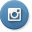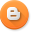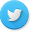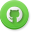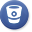## Monday, January 4, 2016

### SUM Using A Formula (SQL)Today I was asked to pull some data and give a total difference of two different columns in a table in SQL.  Then I realized that not everyone would have came across this.  Even though in the past I have showed you how to use the SUM Function in SQL.  This time I thought I would go one step further and show you how to get a SUM while using a formula in SQL.

Use the SUM() Function to get the total of a specific numeric column.  With that being said, the Sum function doesn't need to be a single field.  In this case, you can use a formula.   Kind of like, getting total Profit by subtracting Expense (Column1) from Total Sales (Column2).   Below is the specific syntax to use.

SQL SUM Example:

``````
SELECT  SUM(Column1 - Column2)
FROM TableName;

``````

Please note, you can also preform groupings while using this function as well.  I will show you those at a later date.

If you like this post and want to see more, follow me on my website www.chadcompton.com
Or if you prefer...